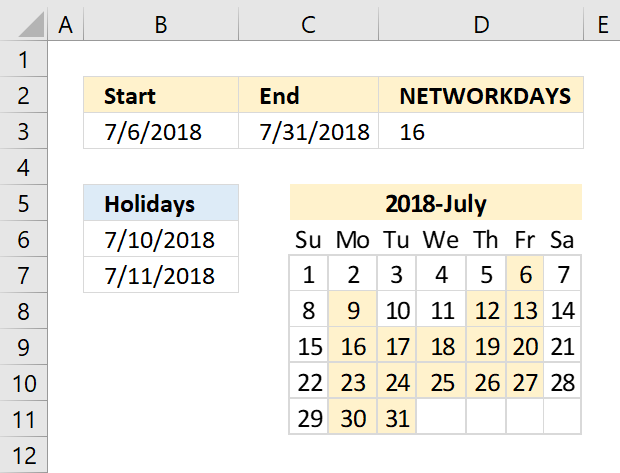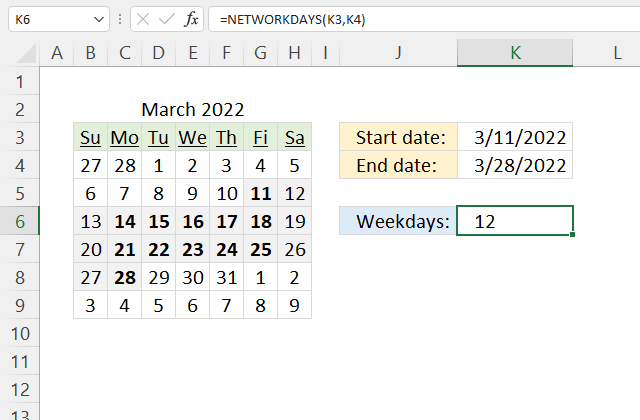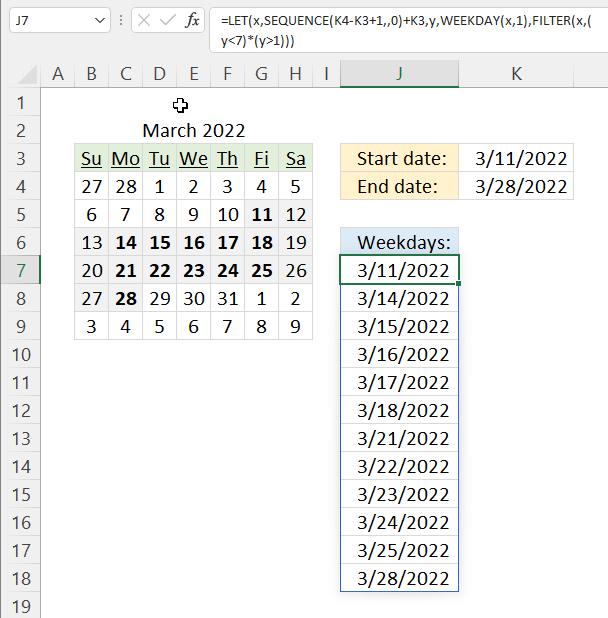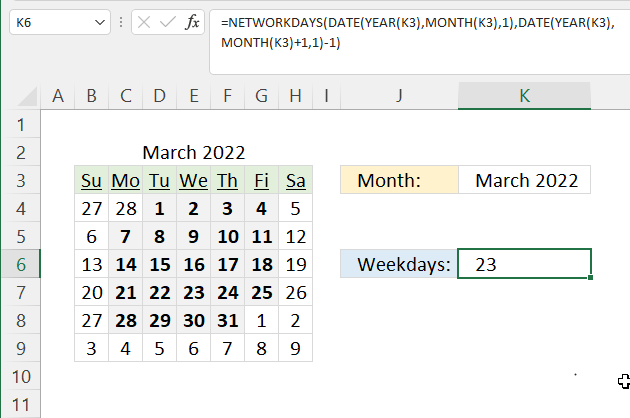Author: Oscar Cronquist Article last updated on May 31, 2022The NETWORKDAYS function returns the number of working days between two dates, excluding weekends. It also allows you to ignore a list of holiday dates that you can specify.

Formula in cell D3:

=NETWORKDAYS(B3,C3, B6:B7)

## 1. Excel Function Syntax

NETWORKDAYS(start_date, end_date, [holidays])

## 2. Arguments

 start_date Required. The start date you want to use in the function. end_date Required. The end date you want to use. [holidays] Optional. Excludes this list of dates from being counted.

## 3. NETWORKDAYS Function exampleThe formula in cell K6 counts weekdays (Monday to Friday) between two dates.

Formula in cell K6:

=NETWORKDAYS(K3, K4)

### Explaining formula

#### Step 1 - NETWORKDAYS function

The NETWORKDAYS function returns the number of working days between two dates, excluding weekends. It also allows you to ignore a list of holiday dates that you can specify.

NETWORKDAYS(start_dateend_date, [holidays])

#### Step 2 - Populate arguments

The NETWORKDAYS function has three arguments, the third is optional.

start_date - K3

end_date - K4

[holidays] - Not used here

#### Step 3 - Evaluate NETWORKDAYS function

NETWORKDAYS(K3, K4)

becomes

NETWORKDAYS(44631, 44648)

and returns 12.

## 4. Create a list of weekdays onlyThis example demonstrates how to extract a list of weekdays (Monday to Friday) or networking days. The formula in cell J7 uses the two dates specified in cells K3 and K4 to create a list of weekdays.

Excel 365 formula:

=LET(x,SEQUENCE(K4-K3+1,,0)+K3,y,WEEKDAY(x,1),FILTER(x,(y<7)*(y>1)))

### Explaining formula

This formula is a dynamic array formula and works only in Excel 365, it is entered as a regular formula

#### Step 1 - Calculate days between dates and add one

The minus and plus signs let you perform arithmetic operations in an Excel formula.

K4-K3+1

becomes

44648-44631+1

equals 18.

#### Step 2 - Create a sequence of numbers from 0 (zero) to 18

The SEQUENCE function creates a list of sequential numbers to a cell range or array. It is located in the Math and trigonometry category and is only available to Excel 365 subscribers.

SEQUENCE(rows, [columns], [start], [step])

SEQUENCE(K4-K3+1,,0)

becomes

SEQUENCE(18,,0)

and returns

{0; 1; 2; 3; 4; 5; 6; 7; 8; 9; 10; 11; 12; 13; 14; 15; 16; 17}.

#### Step 3 - Add start date to sequence of numbers

SEQUENCE(K4-K3+1,,0)+K3

becomes

{0; 1; 2; 3; 4; 5; 6; 7; 8; 9; 10; 11; 12; 13; 14; 15; 16; 17} + 44631

and returns

{44631; 44632; 44633; 44634; 44635; 44636; 44637; 44638; 44639; 44640; 44641; 44642; 44643; 44644; 44645; 44646; 44647; 44648}.

#### Step 4 - Calculate WEEKDAY number

WEEKDAY(SEQUENCE(K4-K3+1,,0)+K3,1)

becomes

WEEKDAY({44631; 44632; 44633; 44634; 44635; 44636; 44637; 44638; 44639; 44640; 44641; 44642; 44643; 44644; 44645; 44646; 44647; 44648},1)

and returns

{6; 7; 1; 2; 3; 4; 5; 6; 7; 1; 2; 3; 4; 5; 6; 7; 1; 2}.

#### Step 5 - Check if weekday number is smaller than 7

Seven is the last weekday in a week and it represents Saturday if the second argument is one. The smaller than character lets you compare values, the result is a boolean value TRUE or FALSE.

WEEKDAY(SEQUENCE(K4-K3+1,,0)+K3,1)<7

becomes

{6; 7; 1; 2; 3; 4; 5; 6; 7; 1; 2; 3; 4; 5; 6; 7; 1; 2}<7

and returns

{TRUE; FALSE; TRUE; TRUE; TRUE; TRUE; TRUE; TRUE; FALSE; TRUE; TRUE; TRUE; TRUE; TRUE; TRUE; FALSE; TRUE; TRUE}.

#### Step 6 - Check if weekday numbers are larger than one

One represents Sunday and we want to identify dates thare equal to Monday to Friday, in other words, networkingdays.

WEEKDAY(SEQUENCE(K4-K3+1,,0)+K3,1)>1

becomes

{6; 7; 1; 2; 3; 4; 5; 6; 7; 1; 2; 3; 4; 5; 6; 7; 1; 2}>1

and returns

{TRUE; TRUE; FALSE; TRUE; TRUE; TRUE; TRUE; TRUE; TRUE; FALSE; TRUE; TRUE; TRUE; TRUE; TRUE; TRUE; FALSE; TRUE}.

#### Step 7 - Multiply arrays

Both values on the same position in the arrays must return TRUE meaning we need to perform AND-logic. The asterisk lets us multiply values in an Excel formula.

TRUE * TRUE = 1
TRUE * FALSE = 0 (zero)
FALSE * FALSE = 0 (zero)

Boolean values have numerical equivalents, TRUE is equal to 1 and FALSE is equal to 0 (zero).

(WEEKDAY(SEQUENCE(K4-K3+1,,0)+K3,1)<7)*(WEEKDAY(SEQUENCE(K4-K3+1,,0)+K3,1)>1)

becomes

{TRUE; FALSE; TRUE; TRUE; TRUE; TRUE; TRUE; TRUE; FALSE; TRUE; TRUE; TRUE; TRUE; TRUE; TRUE; FALSE; TRUE; TRUE} * {TRUE; TRUE; FALSE; TRUE; TRUE; TRUE; TRUE; TRUE; TRUE; FALSE; TRUE; TRUE; TRUE; TRUE; TRUE; TRUE; FALSE; TRUE}

and returns

{1; 0; 0; 1; 1; 1; 1; 1; 0; 0; 1; 1; 1; 1; 1; 0; 0; 1}.

#### Step 8 - Filter dates based on conditions

The FILTER function is a new function available to Excel 365 subscribers. It lets you extract values based on a condition or criteria.

FILTER(arrayinclude, [if_empty])

FILTER(SEQUENCE(K4-K3+1,,0)+K3,(WEEKDAY(SEQUENCE(K4-K3+1,,0)+K3,1)<7)*(WEEKDAY(SEQUENCE(K4-K3+1,,0)+K3,1)>1))

becomes

FILTER({44631; 44632; 44633; 44634; 44635; 44636; 44637; 44638; 44639; 44640; 44641; 44642; 44643; 44644; 44645; 44646; 44647; 44648},{1; 0; 0; 1; 1; 1; 1; 1; 0; 0; 1; 1; 1; 1; 1; 0; 0; 1})

and returns

{44631; 44634; 44635; 44636; 44637; 44638; 44641; 44642; 44643; 44644; 44645; 44648}.

#### Step 9 - Shorten formula

The LET function allows you to name intermediate calculation results which can shorten formulas considerably and improve performance.

LET(name1name_value1calculation_or_name2, [name_value2calculation_or_name3...])

x - SEQUENCE(K4-K3+1, , 0)+K3
y - WEEKDAY(x, 1)

LET(x, SEQUENCE(K4-K3+1, , 0)+K3, y, WEEKDAY(x, 1), COUNT(FILTER(x, (y<7)*(y>1))))

## 5. Count weekdays in a monthFormula in cell K6:

=NETWORKDAYS(DATE(YEAR(K3), MONTH(K3), 1), DATE(YEAR(K3), MONTH(K3)+1, 1)-1)

### Explaining formula

Steps 1 to 3 calculate the first date of the specified month in cell K3. Steps 4 to 6 calculate the last date in the specified month.

#### Step 1 - Calculate year based on date

The YEAR function returns a number representing the year from a given date.

YEAR(date)

YEAR(K3)

becomes

YEAR(44631)

and returns 2022.

#### Step 2 - Calculate month based on date

The MONTH function returns a number representing the month from a given date.

MONTH(date)

MONTH(K3)

becomes

MONTH(44631)

and returns 3.

#### Step 3 - Calculate first date based on year and month

The DATE function returns an Excel date based on a year, month, and day number.

DATE(year, month, day)

DATE(YEAR(K3), MONTH(K3), 1)

becomes

DATE(2022, 3, 1)

and returns 44621. (3/1/2022)

#### Step 4 - Calculate year based on the date

YEAR(K3)

becomes

YEAR(44631)

and returns 2022.

MONTH(K3)+1

becomes

MONTH(44631)+1

and returns 4.

#### Step 6 - Calculate the last date based on year and month

DATE(YEAR(K3),MONTH(K3)+1,1)-1

becomes

DATE(2022, 4, 1)-1

becomes

44652-1

and returns 44651. (3/31/2022)

#### Step 7 - Calculate the number of days between two dates

NETWORKDAYS(DATE(YEAR(K3),MONTH(K3),1),DATE(YEAR(K3),MONTH(K3)+1,1)-1)

becomes

NETWORKDAYS(44621, 44651)

and returns 23.